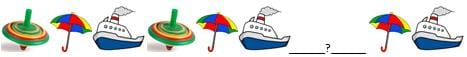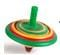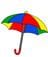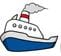Find the missing figure in the given figure p...
Find the missing figure in the given figure pattern.• a)• b)• c)
Can't be determined
• d)### Related TestAavi Vats 16 hours ago
Related Find the missing figure in the given figure pattern.a)b)c)Cant be determinedd)Correct answer is option 'C'. Can you explain this answer?
C part is correct

 1 Crore+ students have signed up on EduRev. Have you?Freak Artworks Jul 08, 2022
Can't be determined

Question Description
This question for Class 1 2022 is part of Class 1 preparation. The Question and answers have been prepared according to the Class 1 exam syllabus. Information about this question covers all topics & solutions for Class 1 2022 Exam. Find important definitions, questions, meanings, examples, exercises and tests below for Find the missing figure in the given figure pattern.a)b)c)Cant be determinedd)Correct answer is option 'C'. Can you explain this answer?.